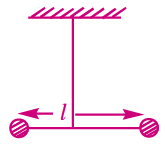Two masses m and m2 are connected at the two ends of a massless rigid rod of length l. The rod is suspended by a thin wire of torsional constant k at the centre of mass of the rod-mass system (see figure). Because of torsional constant k, the restoring torque is τ=kθ for angular displacement θ . If the rod is rotated by θ0 and released, the tension in it when it passes through its mean position will be:

# Two masses m and $\frac{\mathrm{m}}{2}$ are connected at the two ends of a massless rigid rod of length l. The rod is suspended by a thin wire of torsional constant k at the centre of mass of the rod-mass system (see figure). Because of torsional constant k, the restoring torque is $\mathrm{\tau }=\mathrm{k\theta }$ for angular displacement $\mathrm{\theta }$ . If the rod is rotated by ${\mathrm{\theta }}_{0}$ and released, the tension in it when it passes through its mean position will be:1. A

$\frac{3{\mathrm{k\theta }}_{0}^{2}}{\mathrm{l}}$

2. B

$\frac{{\mathrm{k\theta }}_{0}^{2}}{2\mathrm{l}}$

3. C

$\frac{2{\mathrm{k\theta }}_{0}^{2}}{\mathrm{l}}$

4. D

$\frac{{\mathrm{k\theta }}_{0}^{2}}{\mathrm{l}}$

Fill Out the Form for Expert Academic Guidance!l

+91

Live ClassesBooksTest SeriesSelf Learning

Verify OTP Code (required)

### Solution:

From the figure,

$={\mathrm{m\omega }}^{2}{{\mathrm{\theta }}_{0}}^{2}\frac{l}{3}$

$=\mathrm{m}\frac{3\mathrm{k}}{\mathrm{m}{l}^{2}}{{\mathrm{\theta }}_{0}}^{2}\frac{l}{3}=\frac{\mathrm{k}{{\mathrm{\theta }}_{0}}^{2}}{l}$

Hence the correct answer is $\frac{k{{\theta }_{0}}^{2}}{l}.$

## Related content

 Differences & Comparisons Articles in Physics Important Topic of Physics: Reynolds Number Distance Speed Time Formula Refractive Index Formula Mass Formula Electric Current Formula Electric Power Formula Resistivity Formula Weight Formula Linear Momentum Formula+91

Live ClassesBooksTest SeriesSelf Learning

Verify OTP Code (required)